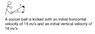# What's the speed of the ball 1 second after it was kicked?

• Melina
In summary, the conversation discusses the initial horizontal and vertical velocities of a soccer ball being kicked, and the speed of the ball 1 second after it was kicked. The correct approach is to first calculate the vertical and horizontal velocities separately, taking into account the acceleration due to gravity, and then use vector addition to find the final speed. The use of trigonometry to calculate angles is unnecessary.f

## Homework Statement

A soccer ball is kicked with an initial horizontal velocity of 15m/s and an initial velocity of 16m/s.
What's the speed of the ball 1 second after it was kicked?

## Homework Equations

Initial velocity = sqrt([horiz. Velocity]^2 + [vertical Velocity]^2)

Final velocity = initial velocity + (gravity*time)

## The Attempt at a Solution

Initial velocity = sqrt (15^2 + 16^2)
Initial velocity = 22m/s

Final velocity = 22m/s + (9.81m/s*1second)
Final velocity = 31.81 m/s

#### Attachments

•20180701_150551.jpg
16.5 KB · Views: 376
Last edited by a moderator:
You are confusing velocity with speed. "Gravity*time" acts on the vertical component of the velocity only, not on the speed.

Speed is distance over time.
I calculated that the distance traveled by the ball is (22sin (2*46.8))/ 9.81 = 49.24 meters

So after one second that the ball was kicked, speed = 49.24m / 1 second = 49.24 m/s
This is still incorrect

Speed is distance over time.
I calculated that the distance traveled by the ball is (22sin (2*46.8))/ 9.81 = 49.24 meters

So after one second that the ball was kicked, speed = 49.24m / 1 second = 49.24 m/s
This is still incorrect

Please explain your work. For instance, what angle are you taking the sine of?

Also we don’t want the distance over time, that gives an average speed. We want the instantaneous speed, I would assume.

I got 46.8 degrees from using the initial horizontal velocity: 15m/s and the vertical velocity: 16m/s.
Theta = tan^-1 (16/15)
Theta = 46.8 degrees

I got 46.8 degrees from using the initial horizontal velocity: 15m/s and the vertical velocity: 16m/s.
Theta = tan^-1 (16/15)
Theta = 46.8 degrees
Why arctangent? You said 16 m/s is the initial speed, not the vertical component.

Either way you don’t need to worry about angles. What is the vertical speed over time? What is the horizontal speed over time? So then what is each after one second?

Melina... In your attempt at a solution in #1 you are doing the vector addition to work out the speed too soon.

Try doing the vector addition after one second.

Final velocity = initial velocity + (gravity*time)
That is true as a vector equation, but later you applied is as though it were a statement about speeds. The 22m/s is not in the vertical direction, but the acceleration is vertical.
You said 16 m/s is the initial speed, not the vertical component.
That was an omission in post #1. The attachment says vertical.

•Nathanael